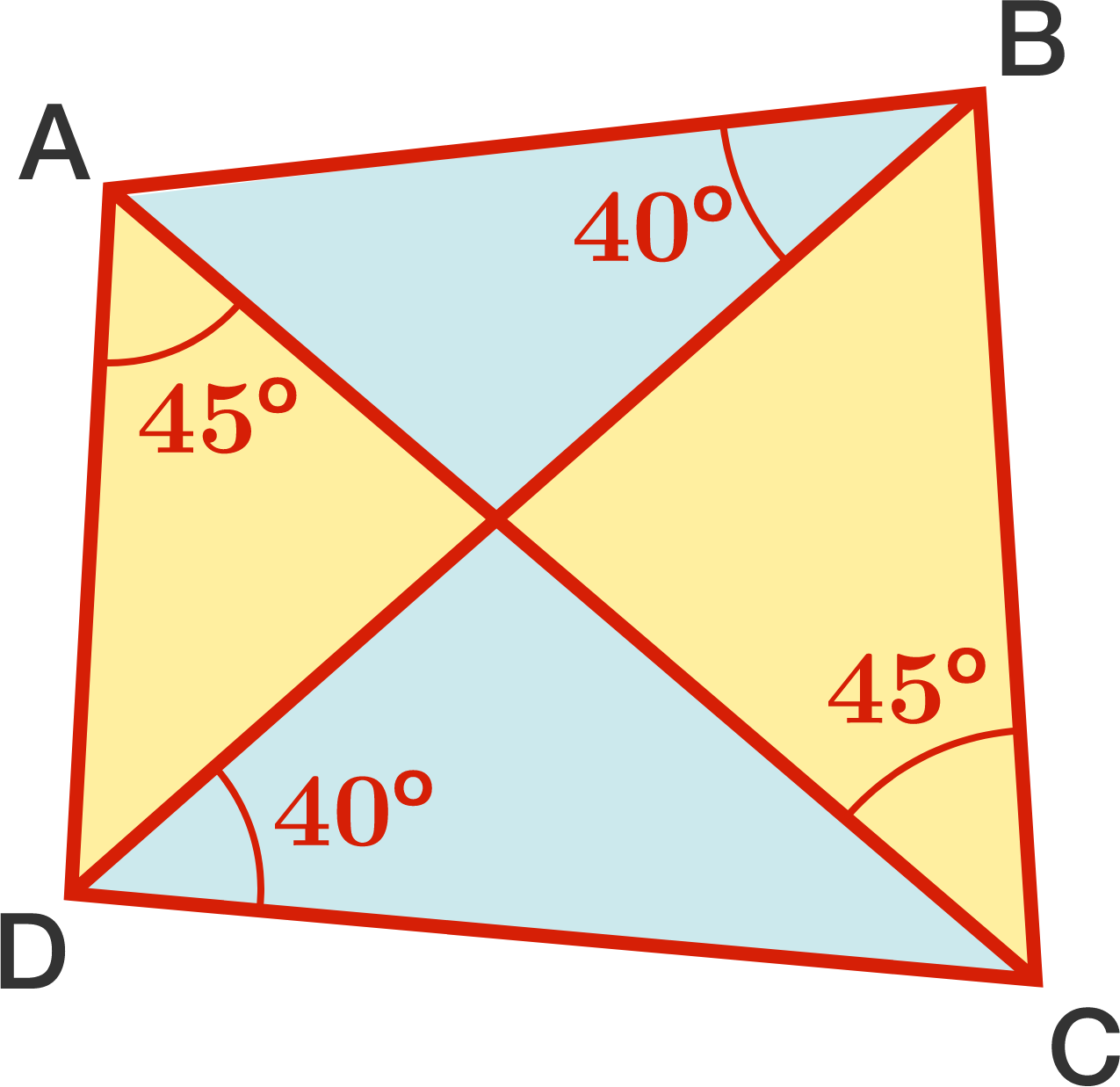# Two-tone PinwheelIn a quadrilateral $ABCD$ in which $\angle ABD=\angle BDC=40^\circ$ and $\angle BCA=\angle CAD=45^\circ$, find the smaller of the two angles between the diagonals.

Report the positive difference between the two solutions to this problem.

×